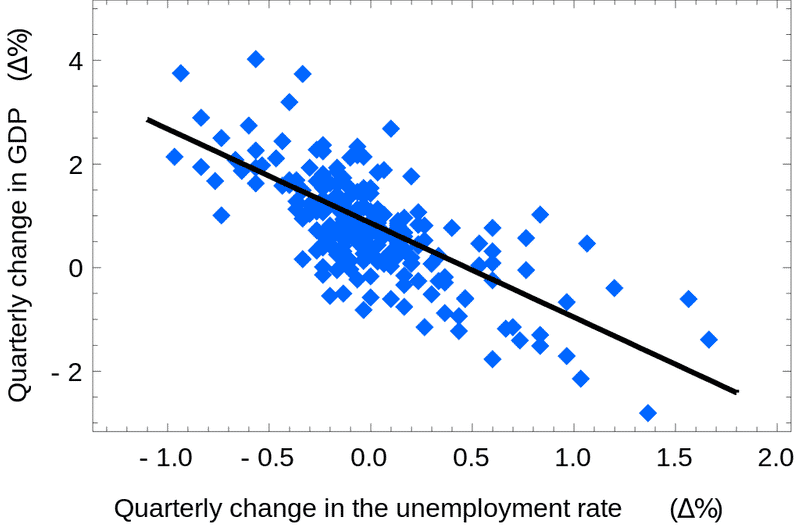# Simple Example of Linear Regression With scikit-learn in Python

Linear Regression is a linear approach which is useful for finding a relationship between predictor or independent variable and response or dependent variable. In simple word, if you change the value of a variable, then it will change another variable value.

So in linear regression, you will always get a different value for another independent variable. With linear regression, we can predict the value of our variable for a given value of the independent variable.

further, you can learn: Fitting large dataset into Linear Regression model

The simple linear regression equation is denoted like this:

f(x) = mx +y

As you can see, it’s an equation of a linear line on a graph where f(x) is the mean or expected value of x for a given value of y, m is the slope of the line and y is the intercept. Below is a picture example:Linear Regression Example – Picture from Wikipedia

In this tutorial, we are going to represent linear regression in Python using the popular scikit-learn library or module. So first, you need to have installed the scikit-learn module. You will able to know how to install the Scikit Learn library from https://scikit-learn.org/stable/install.html.

### Purpose of linear regression in Python

The purpose of linear regression is to predict the data or value for a given data. The given data is independent data which we call as features and the dependent variables are labels or response. IThe main field of using linear regression in Python is in machine learning.

With linear regression, we will train our program with a set of features. By analyzing these features, our program will be able to predict the labels or values for a given set of features. For example, in stock marketing, weather forecasting linear regression use widely.

### Linear regression example with Python code and scikit-learn

Now we are going to write our simple Python program that will represent a linear regression and predict a result for one or multiple data.

In our example, we are going to make our code simpler. So we eliminate to create the plotting graph and only focused on creating a program where we will pass data and it will return the predicted value. I am trying to make the program simpler for better and easy understand and focusing only on the calculation to get the predicted values.

First, let’s import linear_model from scikit-learn library:

`from sklearn import linear_model`

Now take features and labels set to train our program:

```features = [,,,]
labels = [27, 11, 75, 155]```

After that create our model and fit the label and features to our model:

```clf = linear_model.LinearRegression()
clf=clf.fit(features,labels)```

In the end, pass data to the model and print the predicted result:

```predicted = clf.predict([])
print(predicted)```

Now see the complete and final code all together:

```from sklearn import linear_model

features = [,,,]
labels = [27, 11, 75, 155]

clf = linear_model.LinearRegression()
clf=clf.fit(features,labels)

predicted = clf.predict([])
print(predicted)
```

In our program, we have take 8 as the data for which we want to get the predicted result. If we run our program than we will able to see the predicted value. The program actually finds the closest line that will fit closely.

If we want, then we can pass multiple features for which we want to get values like this:

`predicted = clf.predict([, , ])`

We will get predicted values for each feature we provide.

I hope you have understood the example of Python linear example.

### One response to “Simple Example of Linear Regression With scikit-learn in Python”

1.charles owuor says:

In your example, y is used as intercept. this could confuse beginners in regression analysis. y is used as an outcome variable in conventional regression analysis. You could use a different constant.